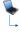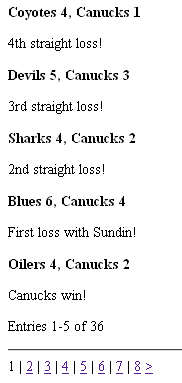# How to paginate the display of MySQL data in PHP

First published on January 18, 2009

Suppose that you have a long list of data rows stored in a MySQL database. This could be something like personal or business contacts, book entries, or blog posts. Without pagination, the display might be hard for a user to manage.

Here’s a PHP code outline to paginate the output of data to show something like this:```<?php

// How many entries you want to show per page
\$perpage = 5;

// Find out what page of entries you are looking for
// The reason why you subtract 1 is so that you can calculate which returned entry (for later when we query the database) to start at
if (isset(\$_GET['page'])) {
\$page = max(intval(\$_GET['page'] - 1), 0);
\$page_original = intval(\$_GET['page']);
}
else {
\$page = 0;
\$page_original = 1;
}

\$start_at = \$page * \$perpage;
\$start_at_public = \$start_at + 1;

mysql_select_db('dbname') or die( 'Unable to select database');

// How many entries are there?
\$query = 'SELECT COUNT(*) FROM `tablename`';
\$result = mysql_query(\$query) or die('Error in query: ' . \$query);
\$total_entries = mysql_result(\$result, 0);

// You've asked for a page that is too high
if (\$total_entries < \$start_at_public) {
die('Not enough entries to go that high.');
}

// Grab the specified number of entries, starting at the appropriate record
\$query2 = "SELECT `title`, `content` FROM `tablename` ORDER BY `publishdate` DESC LIMIT \$start_at, \$perpage";
\$result2 = mysql_query(\$query2);

// Print those entries!
while (\$entries = mysql_fetch_object(\$result2)) {
print '<div class="entry">' . "\n";
print '<h2>' . \$entries->title . '</h2>' . "\n";
print '<p>' . \$entries->content . '</p>' . "\n";
print '</div>' . "\n";
}

mysql_close();

\$end_value_this_page = min(\$start_at + \$perpage, \$total_entries);

if ((\$end_value_this_page == \$total_entries) && (\$start_at_public == \$total_entries)) {
print '<p>Entry ' . \$end_value_this_page . ' of ' . \$total_entries . '</p>' . "\n";
}

else {
print '<p>Entries ' . (\$start_at_public) . '-' . \$end_value_this_page . ' of ' . \$total_entries . '</p>' . "\n";
}

// How many pages of content are there?
\$total_pages = ceil(\$total_entries / \$perpage);

if (\$total_pages != 1) {

print '<hr />' . "\n";

print '<div class="paginator">' . "\n";

// Loop through each of the pages
for (\$page_count = 1; \$page_count <= \$total_pages; ++\$page_count) {

if (\$page_original == \$page_count) {

// Get the "previous" link if there is more than one page and if this isn't the first page
if (\$total_pages > 1 && \$page_count != 1) {
\$previous_link = '<a href="?page=' . (\$page_count - 1) . '"><</a> | ';
}

// If there are more pages than this,  prepare the "next" link
if (\$page_count != \$total_pages) {
\$next_link = ' <a href="?page=' . (\$page_count + 1) . '">></a>';
}
}

else {
\$page_links .= '<a href="?page=' . \$page_count . '">' . \$page_count . '</a>';
}

if (\$page_count != \$total_pages) {
// Print a separator for all pages but the last page